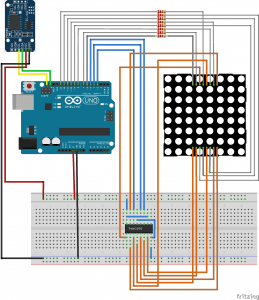26/11/2018

# Arduino Binary Clock

This post is build on top of the LED matrix in the earlier post. I always wanted to build an Binary clock, and since I had the LED matrix ready, and I found a concept done by other people in their project, I had an idea to re-use my LED matrix and turn it into a binary clock.

Here are the list of material that you will need:

• Arduino uno/nano
• Realtime clock DS3231
• Button cell battery CR2032
• 8×8 LED matrix
• 74HC595N shift register

## Step 1. The DesignThe LED matrix consist of 8×8 LED, so we design the top row to be YYMMDD with a space of empty column between the Year and Month and between Month and Date.

The hour is being displayed at the bottom row in the format of HHMMSS. We also allow a space of empty column in between hour and minute and between minute and second.

The Least significant bit is at the bottom and the Most significant bit is at the top. Each digit is being displayed using four LED, acting like BCD (Binary Coded Decimal) code, which is able to count up to 10. However the hour will only count up to 24 and both the minute and seconds will only count up to 59.

## Step 2. The wiring diagramLeveraging the same circuit to connect the LED matrix to Arduino, we only need to at the RTC (Real Time Clock) DS3231 to the Arduino. To do this we are connecting the SCL line from the DS3231 module to SCL line of Arduino and connecting the SDA line from the DS3231 to SDA line of Arduino. Once this is done we then connect the VCC line to 5V and the ground to Arduino GND.

The detail explanation of the other part of the circuit can be found in my previous instructables post related to 8×8 Matrix Letter game. If you would like to know more details please visit the linked page, you can also asked me if you have any questions related to the connection.

Once this is done, we can start to load the code from the Arduino interface. To initialise the RTC module we need to include the following library.

```#include <Time.h>
#include <TimeLib.h>
#include <DS3231.h>```

Then to initialise the clock for the first time, add the following code in the setup section of the code.

```// Set sketch compiling time
clock.setDateTime(__DATE__, __TIME__);```

The above initialisation is to make sure that the DS3231 is sync with the current date and time the first time it is being executed. As long as you had the button cell battery in the RTC clock, it will keep maintaining the clock, and you don’t have to re-initialise it every time.

The following code is the key to displaying the correct binary output:

```int day_one = _day % 10;
int day_ten = _day / 10;
int month_one = _month % 10;
int month_ten = _month / 10;
int year_one = _year % 10;
int year_ten = (_year / 10) % 10;
int sec_one = _second % 10;
int sec_ten = _second / 10;
int min_one = _minute % 10;
int min_ten = _minute / 10;
int hour_one = _hour % 10;
int hour_ten = _hour / 10;```

We need to separate the one digit and the ten digit of the day, month, year, hour, minute and second by using the division and the mod to get the remainder. Then the output is combined together before being pushed out to the LED matrix. This is done in the same procedure called display_time() as below:

```output = day_one << 4 | sec_one;
output = day_ten << 4 | sec_ten;
output = 0;
output = month_one << 4 | min_one;
output = month_ten << 4 | min_ten;
output = 0;
output = year_one << 4 | hour_one;
output = year_ten << 4 | hour_ten;```

The day digit is being combined with the second digit, the month digit is being combined with the minutes digit and the year digit is being combined with the hour digit. once this is done we then called the procedure display_char(output) to display all the combination into the LED matrix.

Final Thoughts

You can see the realtime clock in action in the following video.

You can download the full source code in the following location. If you like this post, please leave a comment and also don’t forget to subscribe to receive frequent update and more similar projects like this one. You can easily expanded this project to be able to display Fibonacci clock. I have some concept in my mind already, so when I had the chance I will make a small modification to be able to display the Fibonacci clock.